• ## C语言程序设计实践-扫雷小游戏

千次阅读 多人点赞 2018-10-09 21:02:16
C语言程序设计实践-扫雷小游戏 大连海事大学 信息科学技术学院 电子信息工程专业四班 C语言程序实训-扫雷小游戏 实验代码 #include&lt;stdio.h&gt; #include&lt;stdlib.h&gt; #include&lt;time...
C语言程序设计实践-扫雷小游戏
大连海事大学 信息科学技术学院 电子信息工程专业四班 C语言程序实训-扫雷小游戏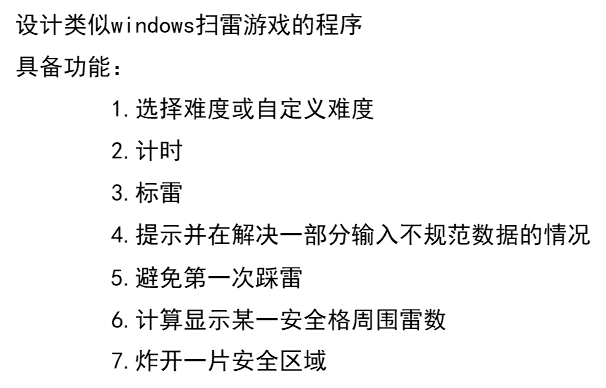实验代码
#include<stdio.h>
#include<stdlib.h>
#include<time.h>
#include <windows.h>
#define xx 51
#define yy 51

int a[xx][yy];
char show[xx][yy];
Int boom[xx][yy];
int hang,lie;
double begin, finish;
int oo;

void menu(void);
void set(int boom[xx][yy]);
void display(char show[xx][yy]);
int sweep(int boom[xx][yy], char show[xx][yy]);
int num(int boom[xx][yy], char show[xx][yy], int x, int y);
void nandu();

主函数如下
int main()
{
int m, n;
kaishi:
for (m = 0; m<xx; m++)
{
for (n = 0; n<yy; n++)
{
show[m][n] = '\0';
boom[m][n] = 0;
a[m][n] = 0;//为数组进行初始化
}
}

menu();
int f;
scanf("%d", &f);
if (f == 1)
{
system("cls");
nandu();
for (m = 0, n = 0; n<lie; n++)//设置边界标识
a[m][n] = 1;
for (m = (hang-1), n = 0; n<lie; n++)//设置边界标识
a[m][n] = 1;
for (m = 0, n = 0; m<hang; m++)//设置边界标识
a[m][n] = 1;
for (m = 0, n = (lie-1); m<hang; m++)//设置边界标识
a[m][n] = 1;

system("cls");
set(boom);
display(show);
sweep(boom, show);
printf("按任意键返回主菜单\n");
scanf("%d", &m);
system("cls");
goto kaishi;
}
return 0;
}

void menu()
{
printf("\n");
printf("                  \n");
printf("      主菜单      \n");
printf("\n");
printf("    1.进入游戏     \n");
printf("    2.退出游戏     \n");
printf("\n");

printf("（输入对应序号进入)\n");
printf("copyright by team 6\n");
printf("\n");
printf("\n");
printf("\n");
}

选择难度
void nandu()//难度调节
{tou:
color(7);
printf("1.简单模式\n");
color(9);
printf("2.普通模式\n");
color(4);
printf("3.困难模式\n");
color(5);
printf("4.噩梦模式\n");
color(7);
printf("5.自定义(行列小于50)\n");
int mm;
scanf("%d", &mm);
switch (mm)
{
case 1:oo = 10; hang = 11; lie = 11; break;
case 2:oo = 40; hang = 18; lie = 18; break;
case 3:oo = 99; hang = 23; lie = 23; break;
case 4:oo = 120; hang = 23; lie = 23; break;
case 5:	printf("行 列 雷\n");
scanf("%d %d %d",&hang,&lie,&oo);
hang=hang+2;
lie=lie+2;
break;
default:{printf("错误,请重新选择");

goto tou;
system("cls");
}
}

}

void set(int boom[xx][yy])
{
int count = oo;
int x = 0;
int y = 0;
srand((unsigned)time(NULL));
while (count)
{
x = rand() % (hang-3) + 1;
y = rand() % (lie-3) + 1;
if (boom[x][y] == 0)
{
boom[x][y] = 1;
count--;
}
}
}

void display(char show[xx][yy])
{
int i = 0;
int j = 0;
printf("  ");
for (i = 1; i<hang - 1; i++)
{
printf(" %2d ", i);
}
printf("\n");
for (i = 1; i < hang - 1; i++)
{

printf("  ");
for (j = 1; j<lie - 1; j++)
{
printf("|---");
}
printf("|\n");
printf("%2d", i);
for (j = 1; j < lie - 1; j++)
{
printf("| %c ", show[i][j]);
}
printf("|\n");
}
printf("  ");
for (j = 1; j < lie - 1; j++)
{
printf("|---", show[i][j]);
}
printf("|\n");
}

int sweep(int boom[xx][yy], char show[xx][yy])
{
int num(int boom[xx][yy], char show[xx][yy], int x, int y);
int x, y, count = 0, l, k, win = 0;

begin = clock();
while (1)
{

start:
win = 0;
for (l = 1; l<hang - 1; l++)
{
for (k = 1; k<lie - 1; k++)
if (show[l][k] >= 48 && show[l][k]<57)//胜利条件检测
win++;

}
if (win == ((hang - 2)*(lie - 2) - oo))//胜利条件判断
break;

printf("请输入坐标,输入坐标的相反数可以标记为雷区\n");
scanf("%d %d", &x, &y);
if (x>(hang-2) || y>(lie-2)||x<-(hang-2)||y<-(lie-2)||!((x>0&&y>0)||(x<0&&y<0)))
{

system("cls");
display(show);
printf("输入错误，请重新输入\n");
goto start;
}

if (x<0)
{
if (show[-x][-y] == '\0')
show[-x][-y] = '!';
else
if(show[-x][-y] == '!')
show[-x][-y] = '\0';
else {goto start;
}
system("cls");
display(show);

continue;
}

if (count == 0)//防止第一次踩雷
{
if (boom[x][y] == 1)//count在这里是计步作用 记录操作次数
{
boom[x][y] = 0;
l = rand() % (hang-3)+ 1;
k = rand() % (lie-3) + 1;
if (boom[l][k] == 0)
{
boom[l][k] = 1;

}

}
}

if (boom[x][y] == 1){
for (l = 1; l<hang - 1; l++){
for (k = 1; k<lie - 1; k++)
if (boom[l][k] == 1)
show[l][k] = '*';//失败时雷区显示
}
system("cls");
display(show);
finish = clock();
printf("你踩到地雷了！\n");
printf("游戏结束,用时%d秒\n", (int)(finish - begin) / 1000);
return 0;//游戏失败
}
else
{
num(boom, show, x, y);
system("cls");
display(show);
count++;

}

}
finish = clock();
printf("你赢了!!\n");
printf("游戏结束,用时%d秒\n", (int)(finish - begin) / 1000);
printf("输入任意数字返回主菜单\n");
return 0;

}

int num(int boom[xx][yy], char show[xx][yy], int x, int y)//查看一点周围雷的个数

{
int nnn = 0, p, q;
if (a[x][y] == 0)
{

if (boom[x - 1][y - 1] == 1)
nnn++;
if (boom[x - 1][y] == 1)
nnn++;
if (boom[x - 1][y + 1] == 1)
nnn++;
if (boom[x][y - 1] == 1)
nnn++;
if (boom[x][y + 1] == 1)
nnn++;
if (boom[x + 1][y - 1] == 1)
nnn++;
if (boom[x + 1][y] == 1)
nnn++;
if (boom[x + 1][y + 1] == 1)
nnn++;
show[x][y] = nnn + '0';
if (nnn == 0){//连锁反应递归
a[x][y] = 1;

for (p = x - 1; p<x + 2; p++)
{
for (q = y - 1; q<y + 2; q++)
num(boom, show, p, q);

}

}
}

else return 0;


运行结果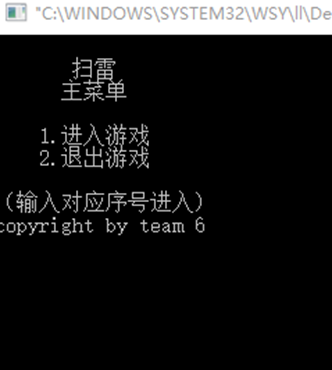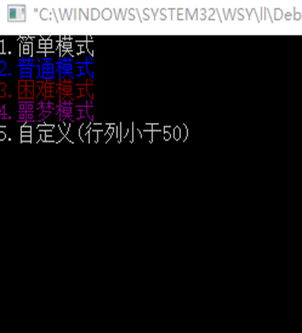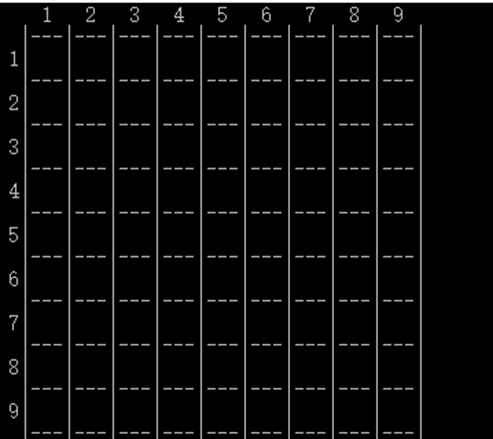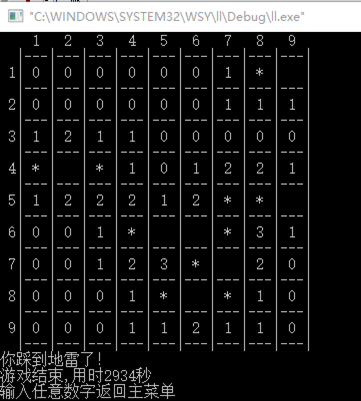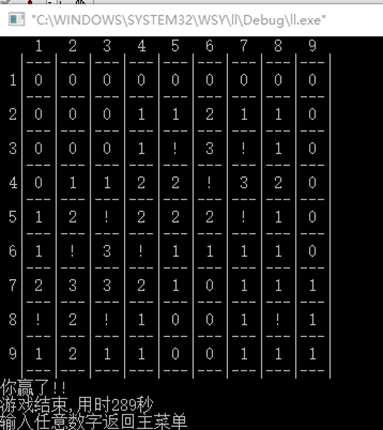实验心得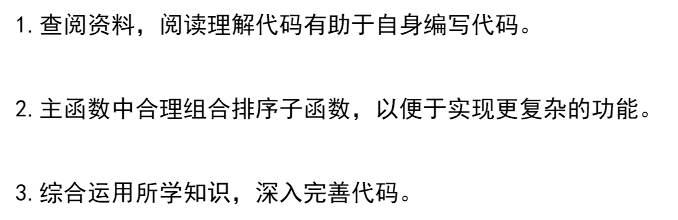COPYRIGHT BY LIDONGLIN



展开全文• 以下是游戏截图：迷宫示意图说明：0是玩家所在位置，#号是障碍物，玩家不可以穿越，玩家只可以移动到空白的地方，例如按s往下后出现这种情况如图所示我们发现0即玩家已经往下走了一格另外只能输入w、s、a、d这四个...
以下是游戏截图：迷宫示意图说明：0是玩家所在位置，#号是障碍物，玩家不可以穿越，玩家只可以移动到空白的地方，例如按s往下后出现这种情况如图所示我们发现0即玩家已经往下走了一格另外只能输入w、s、a、d这四个按键，输入其他无反应。代码如下：#include #define ROW 6#define COL 6int main(intargc, const char * argv[]) {char map[ROW][COL]={{'#','#','#','#','#','#'},{'#','0','#','#',' ',' '},{'#','','#','#',' ','#'},{'#','',' ','#',' ','#'},{'#','#',' ',' ',' ','#'},{'#','#','#','#','#','#'}};char input;//表示人的位置int x = 1;int y = 1;while (1) {// 打印for (int i = 0; i < ROW;  i++) {for (int j = 0; j< COL; j++) {printf("%c", map[i][j]);}printf("\n");}// 胜利的代码if (x == 0|| x == ROW - 1|| y == 0 || y == COL- 1) break;// 提示用户的输入printf("请输入 w(上) s(下) a(左) d(右)\n");// 输入数据, 判断正确while (1){printf("> ");input = getchar();while (getchar() != '\n');if (input =='w' || input == 's' || input == 'a' || input =='d') {break;}}// 更新地图switch (input) {case 'W':case 'w':// 判断下一个位置是否可以走, 如果可以走就交换地图中的数据// 如果不能走就不变// 即 判断 (x - 1, y) 是不是空格, 如果是空格就可以走if (map[x- 1][y] == ' '){// 交换int  temp = map[x - 1][y];map[x -1][y] = map[x][y];map[x][y] = temp;// 交换后更新 x yx--;}break;case 's':if (map[x+ 1][y] == ' '){// 交换int  temp = map[x + 1][y];map[x +1][y] = map[x][y];map[x][y] = temp;// 交换后更新 x yx++;}break;case 'a':if (map[x][y - 1] == ' ') {// 交换int  temp = map[x][y - 1];map[x][y -1] = map[x][y];map[x][y] = temp;// 交换后更新 x yy--;}break;case 'd':if  (map[x][y + 1] == ' ') {// 交换int   temp = map[x][y + 1];map[x][y +1] = map[x][y];map[x][y] = temp;// 交换后更新 x yy++;}break;}// 清屏system("clear");}printf("恭喜你重获自由...\n");return 0;}
展开全文• 游戏开发 c语言 程序设计 飞机大战。 大一下学期看了知乎上童晶老师的书《“c语言开发设计与游戏开发实践”》，看到飞机大战 那部分兴致大增，遂决定自己也来写一个。 程序用的是单纯的C语言，调用了EasyX库的函数...
• 利用c语言实现的2048小游戏。 可以用来当做结课项目，毕业设计代码注释写的很详细，保证各位可以看懂。 程序的主要使用的相关数据类型：2维数组，枚举，指针 程序运行界面如下图： 开始时随机生成 结束 程序...
利用c语言实现的2048小游戏。
可以用来当做结课项目，毕业设计，代码注释写的很详细，保证各位可以看懂。
程序的主要使用的相关数据类型：2维数组，枚举，指针

程序运行界面如下图：
开始时随机生成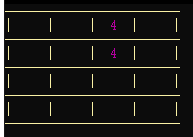结束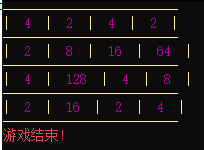程序部分代码实现：
void priMap();  //绘制图形
Dir getNextDirection(); //从键盘读入下一个用户操作
bool canMove(Dir direction); //判断是否可以进行指定方向的操作
void doAction();   //游戏事件
void move(Dir direction);  //移动数字
void New();    //放入一个新的数字
int main() //主函数

void New(){

//为了方便操作,临时存储一下所有空闲格子的指针,这样可以用一个线性的内存随机访问实现对所有空位中任一空位的随机访问.

int* boxes={NULL};
//用来临时保存目标格子的地址
int* target;
//统计一共有多少个有效空格
int count=0;
int i,j;
// color(2);
//统计空位,发现空位即保存地址并累加计数器

for(i=0;i<4;i++)

for(j=0;j<4;j++)

if(!MAP[i][j]){

boxes[count]=&MAP[i][j];

count++;

}
if(count){
//如果有空位,那么对这一位进行随机赋值操作,对于每一位可能性是相同的

target=boxes[rand()%count];
//50%可能出现2 50% 可能出现4

*target=rand()%2?2:4;
}
}

下载链接：
2048小游戏下载地址


展开全文指针 c++
• C语言课程设计报告-猜数游戏C语言课程设计报告-猜数游戏|c语言程序代码编程小程序设计|c语言课程设计报告课程案例#include#includeint dif_1();int dif_2();void menu_1();int menu_in();int dif_1(){int dif_1;dif_...
C语言课程设计报告-猜数游戏C语言课程设计报告-猜数游戏|c语言程序代码编程小程序设计|c语言课程设计报告课程案例#include#includeint dif_1();int dif_2();void menu_1();int menu_in();int dif_1(){int dif_1;dif_1=random(10);return dif_1;}int dif_2(){int dif_2;dif_2=random(100);return dif_2;}void menu_1(){int i;clrscr();for(i=0;i<8;i++) printf("\n");printf("                          Difficuty Choose\n");printf("\n\n");printf("                        1--Between 1 and 10\n\n");printf("                        2--Between 1 and 100\n\n");printf("                        3--Exit\n\n");}int menu_in(){int choose;printf("                            Choose:");scanf("%d",&choose);return choose;}void test(int gess){int num=-1,sum=0;while(num!=gess){printf("Please input number:");scanf("%d",&num);if(num>gess) {printf("high\n");sum++;}else if(numelse printf("Good! You use %d\n",sum);}getchar();getchar();}void error(){printf("Choose error!");getchar();getchar();}main(){int choose;menu_1();choose=menu_in();switch(choose){case 1: test(dif_1());break;case 2: test(dif_2());break;case 3: exit(0);break;default: error();}}
展开全文• 第一篇 c语言基础 第一章：踏上征程前的思想动员 1.1为什么选择c语言 1.2如何写好c语言 1.3语言概述 1.4程序开发周期 1.5VC++、C++、C和TC-认识开发环境和语言的区别 ...第二十二章：c语言程序课程设计游戏 ... ...
• 本文实例为大家分享了C语言实现三子棋小游戏的具体代码，供大家参考，具体内容如下设计思路三子棋的C语言设计的思路：1.设计一个界面：让玩家运行后即可以看到一个界面，而此时界面需要一个让玩家选择的语句，即可以...
• 分享一些个人C语言程序代码[大一上集合] 突然想整理下之前写的一些程序 2019.9-2020.1 大一上学期的更全面的作品的介绍和展示 慢慢填坑吧 1. C语言实现五子棋 代码：【待更新】 介绍：【C语言】用c语言实现简单...
• Problem Description A group of K friends is going to see a movie. However, they are too late to get good tickets, so they are looking for a good way to sit all nearby. Since they are all science ...Golang erlang r语言
• 介绍：运用C语言的数组等相关知识：实现一个简易的三子棋小游戏：三子棋小游戏的大概内容为此游戏在设计时：总共设计了三个文件：game.h: 类型的声明和函数的声明game.c：函数功能的具体实现test.c: 测试文件二....
• 用最简单的Tc3.0编成的小游戏，轻松应对课程设计！还有很多有用的源程序代码
• C语言制作的课程设计小游戏，飞机大战。和团队一起设计并实现该游戏一方面巩固课堂学到的知识，学以致用，加深理论知识的理解，另一方面也锻炼了自己的实际编码能力和团队协作的能力，是一次难得又宝贵的实训开发...
• 题目：家庭财务管理小程序 1.程序分析： 2.程序代码： /*money management system*/ #include "stdio.h" #include "dos.h" main() { FILE *fp; struct date d; float sum,chm=0.0; int len,i,j=0; int...
• 湖南科技大学2018年C语言程序设计实验题——问题 A: 开关灯 题目描述 晚上，Hardware 爸爸正在日光灯映照下的电脑前写代码，突然屋外传来急促的脚步声，他的儿子Mouse跑进来一边高声喊道，“爸爸快把眼睛闭上，...
• C语言编写的黑白棋游戏小程序，包含源代码 需求分析 流程图
• 168 设计口令程序 169 程序自我保护 第六部分 常见试题解答篇 170 水果拼盘 171 小孩吃梨 172 删除字符串中的特定字符 173 求解符号方程 174 计算标准差 175 求取符合特定要求的素数 176 统计...
• 扫雷小游戏作为初学c语言的小白有很大的帮助，其中用到了函数的定义，函数的声明，循环语句，数组思想等等知识，对于代码理解和设计代码思路有很大的帮助，本文就详细介绍了代码的各个步骤和运行结果。希望给到和我...
• 基于模块化的设计思想，想要设计一个三子棋游戏，可以用不同的文件存放不同的代码，通过函数的调用，以体现C语言的面向过程特点。 将程序的源码用于三个文件存放，分别为game.h、game.c和test.c game.h：用于...
• 利用c语言实现的2048小游戏。可以用来当做结课项目，毕业设计，就是比较简单，代码注释写的很详细，保证各位可以看懂。 程序的主要使用的相关数据类型：2维数组，枚举，指针
• 在大一上程序设计基础课期末的时候，想着能不能做个游戏玩玩。一开始打算写象棋，后来还是觉得推箱子更经典一点。由于当时水平太差，还用的是DEV，结果肝了一天一夜才赶出来。。。各位将就着看，就图一乐就行。 代码...
• 前言 ...程序设计流程 数据设计 辅助宏定义：游戏地图的宽和高，以及蛇的最大长度 食物结构体：食物在地图上x和y上的显示 蛇结构体：蛇的长度，蛇的每一节的坐标存储，蛇的移动速度 辅助...贪吃蛇
• C语言编写的贪吃蛇小游戏，这里有完整的代码、截屏和分段程序功能说明。
• 摘自书《C语言课程设计案例精编》 ...在仿Windows应用程序篇中，我们介绍了万年历、画图板、电子时钟、简易计算器、文本编辑器五个应用程序设计与实现。本书从实用性和先进性出发，内容组织合理、通俗易懂。
• 本资源是针对C语言初学者的期末课题设计，采用C语言编写的一个小程序，通过循环、选择、调用等一系列C语言基础语句，实现了一段踢足球的动画，代码简单易懂，运行效果良好，供大家学习与参考
• 笨鸟先飞小游戏编程教学 ...//笨鸟先飞原创小游戏代码 #include <graphics.h> #include<stdio.h> #include<stdlib.h> #include<windows.h> // 函数外全局变量定义 int birdx,birdy;//小鸟
• 这里写目录标题简介一、学习交流二、适合刚学C语言的朋友三、游戏设计思路代码核心一、数据的存储和表示（Data）：二、游戏场景（Scene）：完整代码 简介 一、学习交流 这个程序时我上高中时写出来的，现在已经大一...
• 现在做一个分糖果游戏，从1号朋友开始，将自己的糖果平均分成三份（如果有多余的糖果，则自己立刻吃掉），自己留一份，其余两份分给相邻座位的两个朋友。接着，2号、3号朋友也同样这么做。请问一轮后，每个...
• 本次扫雷小游戏用多文件来编写。首先，要自定义的一个头文件 mine.h，里面有扫雷程序里面所需要的头文件、常量和所有函数的声明。其次，创建主函数 test.c，用自己喜欢的方式构建一个游戏的框架，最后，创建自定义......

# c语言程序设计小游戏代码c语言 订阅